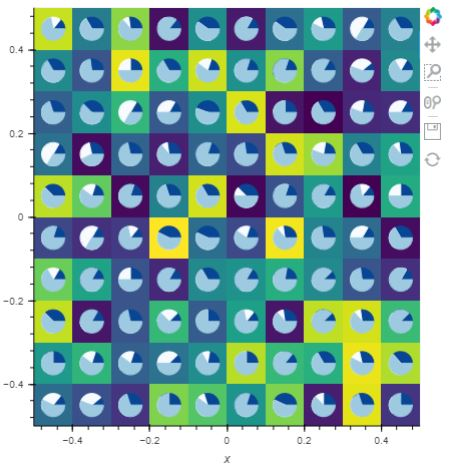# Draw a set of pie charts on one plot

Hello! I am trying to draw a set of pie charts on one plot using wedge. My code is next:

``````import numpy as np
from bokeh.plotting import figure, show

p = figure(plot_height=350)
p.wedge(x=[0, 3], y=[0, 3], radius=[0.1, 0.1], start_angle= [,np.array([1,0])],
end_angle=[[2*np.pi],np.array([2*np.pi,1])], color=["red",["blue","yellow"]])
show(p)
``````

If the second pie chart has only one division, then I can see them both on the plot, but when I divide it on segments, it disappears, could you tell me where I am wrong?

@JhonnyKokos I don’t really understand for sure what you are trying to achieve. But one immediate thing wrong is the nesting in your data, e.g:

``````start_angle= [,np.array([1,0])]
``````

This should just be a single flat 1-dimensional array with the values for all the wedges you want to draw. You will also need to update the `x` and `y` values. All of the properties need exactly as many values as there are wedges (or else a single, constant value for all wedges). If you want to draw 8 wedges then all the lists need to be 8 elements long.

There is also a pie chart example you can refer to here:

pie_chart.py — Bokeh 2.3.0 Documentation

BTW you can certainly draw multiple pie charts with a single call to `wedge` but you might find it simpler to make separate calls to `wedge` for each different chart.

Thank you for the answer, Bryan!

I actually would like to create the grid of pie charts upone the Image object. Something like that:Here is my code:

``````from bokeh.plotting import figure, show
import holoviews as hv
import numpy as np
import panel as pn
from holoviews.streams import Pipe, Buffer

hv.extension('bokeh')

pipe = Pipe(data=np.random.rand(10,10))
n = 10
def hook(plot, element):
for i in range(n):
for j in range(n):
data = np.random.randint(1,5,size=(3))
angle = data/data.sum() * 2*np.pi
plot.state.wedge(x=-.45+i/10, y=-.45+j/10, radius=0.03, start_angle= np.insert(angle[:-1], 0, 0, axis=0),
end_angle=np.cumsum(angle), color=['#084594', '#f7fbff', '#9ecae1' ])

_Image = hv.DynamicMap(hv.Image, streams=[pipe]).opts(hooks=[hook], cmap="viridis", xlim=(-.5, .5), ylim=(-.5, .5), width = 500, height=500)
panel  = pn.Row(_Image)
panel
``````

The problem is that it is too slow with a big number of pie charts (more then 100) I guess in my approach I use “separate calls”, maybe you could pitch me an idea for optimizing my implementaiton? Cause I thought it was possible to create a grid of pie charts in one wedge() function

@JhonnyKokos You can definitely draw all the individual pie chart with one call to `wedge`. The key to note is what I said above: all the arrays for the wedge properties must be flat, one-dimensional arrays, and be the same length.

Every wedge has its own `x`, `y`, `start_angle`, `end_angle`. The arrays for those properties need to have all the values for every wedge you want to draw (and of course, in the same relative order: the first element of each array should be the values for the first wedge, and so).

This is no different at all from how you would pass arrays of `x`, `y`, and `size` to a `scatter` plot to draw 1000 circles or whatever. In fact, it it makes things easier to think about: `wedge` is really a function for making a scatter or wedge glyphs.

1 Like

Thank you very much, @Bryan . You’ve helped me a lot!